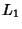## Lemoine Line

The Lemoine line, also called the Lemoine Axis, is the perspectivity axis of a Triangle and its Tangential Triangle, and also the Trilinear Polar of the Centroid of the triangle vertices. It is also the Polar ofwith regard to its Circumcircle, and is Perpendicular to the Brocard Axis.

The centers of the Apollonius Circles,, andare Collinear on the Lemoine Line. This line is Perpendicular to the Brocard Axisand is the Radical Axis of the Circumcircle and the Brocard Circle. It has equationin terms of Trilinear Coordinates (Oldknow 1996).

See also Apollonius Circles, Brocard Axis, Centroid (Triangle), Circumcircle, Collinear, Lemoine Circle, Lemoine Point, Polar, Radical Axis, Tangential Triangle, Trilinear Polar

References

Johnson, R. A. Modern Geometry: An Elementary Treatise on the Geometry of the Triangle and the Circle. Boston, MA: Houghton Mifflin, p. 295, 1929.

Oldknow, A. The Euler-Gergonne-Soddy Triangle of a Triangle.'' Amer. Math. Monthly 103, 319-329, 1996.